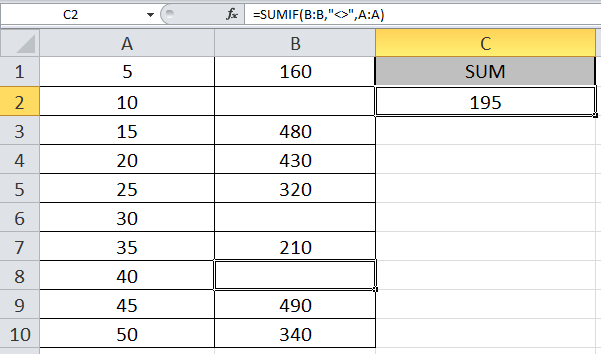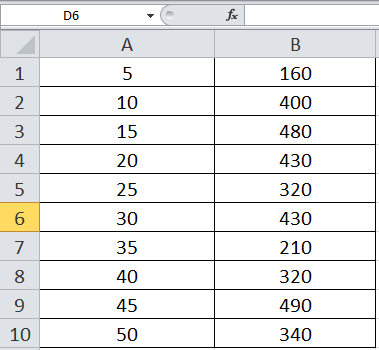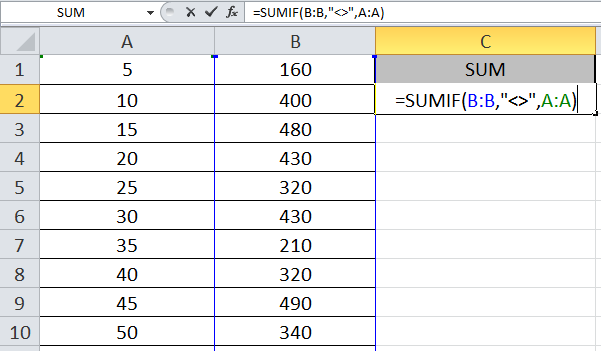# How to Sum Values Based on the Criteria of a Non-Empty Adjacent CellIn this article, we will learn How to Sum Values Based on the Criteria of a Non-Empty Adjacent Cell.

SUM range and Criteria range in SUMIF function

In Excel, Sometimes you might be confused with what is sum range and criteria range. Sum range is where sum is needed and criteria range is where some condition is fulfilled. For example finding the sum of salaries of employees who belong to California. Here sum range is salary and criteria range is State. Another example If we need to find the sum of salaries of employees whose salary is above 50000. Here sum range and criteria range is the same. Let's understand how we can use empty cell criteria in criteria.

Sum if with non empty cells in Excel

SUMIF Excel function gets the sum of range based on the condition and returns the recorded values.

Syntax:

 =SUMIF(cri_range, <> , sum_range)

cri_range : Criteria range, range where criteria is applied

<> : to find not empty only

sum_range : sum range, range where sum is evaluated

Example :

All of these might be confusing to understand. Let's understand how to use the function using an example. we want a formula preferably SUMIF function to sum the numbers in column A only if the corresponding cell in column B is not empty. I have tried to use sumif multiple columns based on non-blank cells.So write the formula where you want to get the sum

Use the formula:

 =SUMIF(B:B,"<>",A:A)

Explanation:

The function checks the cells which are not blank and excel if the cell is not blank, it records the value of the corresponding cell. The function returns the sum of the recorded values.

Sum of values in Column A is done, a condition that the corresponding Column B should not be blank.Press Enter to get the desired result.Now we will remove some of the numbers from Column B.As you can see, as I removed some numbers, the sum changed. The Formula catches the blank cells and considers only the non-blank cells in Column B and returns the sum of corresponding values in Column A.

Here are all the observational notes using the formula in Excel.
Notes :

1. The formula only works with only to look up the exact match.
2. The SUMPRODUCT function considers non-numeric values as 0s.
3. The SUMPRODUCT function considers logic value TRUE as 1 and False as 0.
4. The argument array must be the same size else the function returns an error.
5. SUMPRODUCT function returns the value corresponding to the TRUE values in the returned array after taking individual products in the corresponding array.
6. Operators like equals to ( = ), less than ( < ), less than equal to ( <= ), greater than ( > ), greater than equal to ( >= ) or not equals to ( <> ) can be performed within a formula applied, with numbers only.

Hope this article about How to Sum Values Based on the Criteria of a Non-Empty Adjacent Cell is explanatory. Find more articles on summing values and related Excel formulas here. If you liked our blogs, share it with your friends on Facebook. And also you can follow us on Twitter and Facebook. We would love to hear from you, do let us know how we can improve, complement or innovate our work and make it better for you. Write to us at info@exceltip.com.

Related Articles :

Sum by OFFSET groups in Rows and Columns : The OFFSET function can be used to sum group of cells dynamically. These groups can be anywhere in the sheet.

SUM if date is between : Returns the SUM of values between given dates or period in excel.

Sum if date is greater than given date: Returns the SUM of values after the given date or period in excel.

2 Ways to Sum by Month in Excel: Returns the SUM of values within a given specific month in excel.

How to Sum Multiple Columns with Condition: Returns the SUM of values across multiple columns having condition in excel

Use INDEX and MATCH to Lookup Value : The INDEX-MATCH formula is used to lookup dynamically and precisely a value in a given table.  This is an alternative to the VLOOKUP function and it overcomes the shortcomings of the VLOOKUP function.

How to use wildcards in excel : Count cells matching phrases using the wildcards in excel.

Count Cells that contain specific text: A simple COUNTIF function will do the magic. To count the number of multiple cells that contain a given string we use the wildcard operator with the COUNTIF function.

Excel REPLACE vs SUBSTITUTE function: The REPLACE and SUBSTITUTE functions are the most misunderstood functions. To find and replace a given text we use the SUBSTITUTE function. Where REPLACE is used to replace a number of characters in string…

Popular Articles :

How to use the IF Function in Excel : The IF statement in Excel checks the condition and returns a specific value if the condition is TRUE or returns another specific value if FALSE.

How to use the VLOOKUP Function in Excel : This is one of the most used and popular functions of excel that is used to lookup value from different ranges and sheets.

How to use the SUMIF Function in Excel : This is another dashboard essential function. This helps you sum up values on specific conditions.

How to use the COUNTIF Function in Excel : Count values with conditions using this amazing function. You don't need to filter your data to count specific values. Countif function is essential to prepare your dashboard.

1.